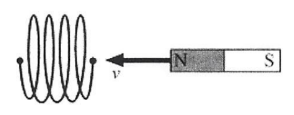Problem: While a magnet is moved toward the end of a solenoid (as shown in the Figure below), a voltage difference is induced between the two ends of the solenoid wire. The voltage difference would be larger if A) the speed of the magnet were increased. B) The bar magnet produced a stronger magnetic field. C) the solenoid contained more loops (while having the same length ). D) All of the above statements are true. E) Only two of the above statements are true.

98% (33 ratings)
Problem Details

While a magnet is moved toward the end of a solenoid (as shown in the Figure below), a voltage difference is induced between the two ends of the solenoid wire. The voltage difference would be larger if

A) the speed of the magnet were increased.

B) The bar magnet produced a stronger magnetic field.

C) the solenoid contained more loops (while having the same length ).

D) All of the above statements are true.

E) Only two of the above statements are true.